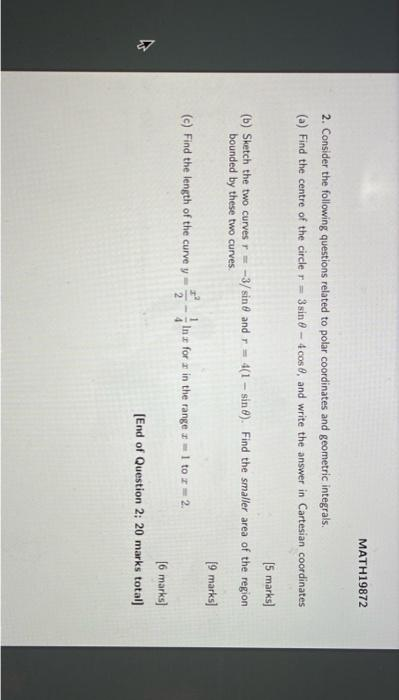# (Solved): 2. Consider the following questions related to polar coordinates and geometric integrals. (a) Find ...2. Consider the following questions related to polar coordinates and geometric integrals. (a) Find the centre of the circle $$r=3 \sin \theta-4 \cos \theta$$, and write the answer in Cartesian coordinates [ 5 marks] (b) Sketch the two curves $$r=-3 / \sin \theta$$ and $$r=4(1-\sin \theta)$$. Find the smaller area of the region bounded by these two curves. $$[9$$ marks] (c) Find the length of the curve $$y=\frac{x^{2}}{2}-\frac{1}{4} \ln x$$ for $$x$$ in the range $$x=1$$ to $$x=2$$. [6 marks] [End of Question 2; 20 marks total]

We have an Answer from Expert